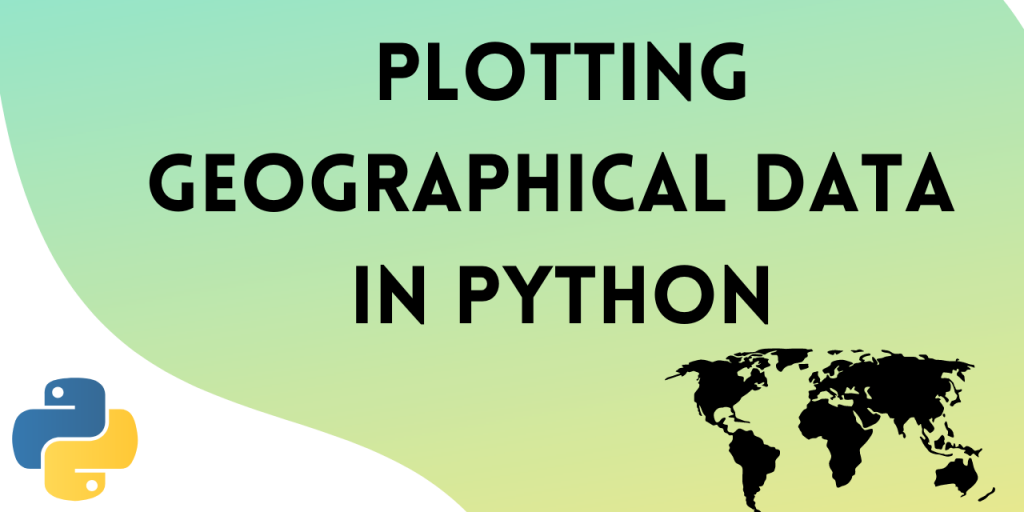# Plot Geographical Data on a Map Using Python PlotlyIn this tutorial, we will learn how to plot geographical data on a map using Python Plotly. For this demonstration, we’ll plot COVID-19 cases from ourworldindata.org dataset.

## Steps to Plot Geographical Data on a Map in Python

Let’s get started.

### 1. Import the required libraries

Let’s start with importing the necessary libraries. We need to import the following two libraries:

```import pandas as pd
import plotly.express as px
```

```!wget https://covid.ourworldindata.org/data/owid-covid-data.csv
```

Output :

```df = pd.read_csv('owid-covid-data.csv')
```

### 3. Plotting the COVID-19 Dataset on a Map

Now we can use Plotly to plot the data from the dataset above.

We are plotting a Choropleth Map. It is a map which uses differences in shading, colouring, or the placing of symbols within predefined areas to indicate the average values of a particular quantity in those areas.

We are going to plot the number of new cases each day. Plotly lets us do that through animations.

Here’s the code to plot the colors and shades on the map:

```fig = px.choropleth(df, locations="iso_code",
color="new_cases",
hover_name="location",
animation_frame="date",
title = "Covid Cases plotted using Plotly",                 color_continuous_scale=px.colors.sequential.PuRd)

fig.show()
```

Output :

The output shows how the map looks over three different months of the year. We can see that the virus was spreading rapidly in China in the month of February, in USA in the month of April and in India in the month of September.

You can also hover over any region of the map and view the number of new cases.

Plotly animations make it convenient to visualize time series data.

### 4. Complete code to Plot Geographical Data using Python Plotly

The complete code for this section in given below :

```import pandas as pd
import plotly.express as px

!wget https://covid.ourworldindata.org/data/owid-covid-data.csv

#import dataset

#plot
fig = px.choropleth(df, locations="iso_code",
color="new_cases",
hover_name="location",
animation_frame="date",
title = "Covid Cases plotted using Plotly",                 color_continuous_scale=px.colors.sequential.PuRd)

fig.show()

```

### 6. Plotting COVID-19 Asia Data

You can also set the scope of the map to Asia. Let’s see how to plot geographical data for the content of Asia.

To set the scope of the plot to Asia, set the parameter ‘scope‘ to ‘asia’.

```import pandas as pd
import plotly.express as px

!wget https://covid.ourworldindata.org/data/owid-covid-data.csv

#import dataset

#select entries with the continent as asia
df = df[df.continent == 'Asia']

#plot
fig = px.choropleth(df, locations="iso_code",
color="new_cases",
hover_name="location",
animation_frame="date",
title = "Daily new COVID cases",
scope ='asia',  color_continuous_scale=px.colors.sequential.PuRd)